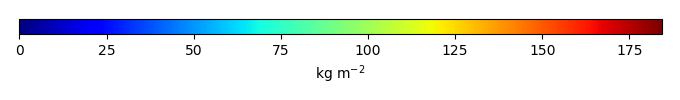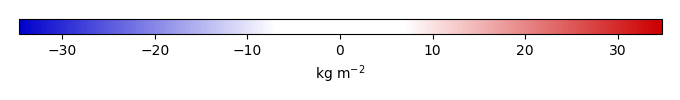# Mean State

Period Mean (original grids) [Pg]
Model Period Mean (intersection) [Pg]
Model Period Mean (complement) [Pg]
Benchmark Period Mean (intersection) [Pg]
Benchmark Period Mean (complement) [Pg]
Bias [kg m-2]
Bias Score 
Spatial Distribution Score 
Overall Score 
Benchmark [-] 34.1
CLM4 [-] 31.5 32.8 0.148 33.8 0.315 0.0325 0.65 0.89 0.77
CLM4.5 [-] 38.1 38.5 0.300 33.8 0.315 1.53 0.60 0.77 0.68
CLM5 [-] 41.4 42.1 0.279 33.8 0.315 2.30 0.62 0.80 0.71
Period Mean (original grids) [Pg]
Model Period Mean (intersection) [Pg]
Model Period Mean (complement) [Pg]
Benchmark Period Mean (intersection) [Pg]
Benchmark Period Mean (complement) [Pg]
Bias [kg m-2]
Bias Score 
Spatial Distribution Score 
Overall Score 
Benchmark [-] 44.8
CLM4 [-] 22.1 20.8 0.00819 44.4 0.478 -3.43 0.59 0.89 0.74
CLM4.5 [-] 17.6 16.7 0.0228 44.4 0.478 -4.03 0.53 0.76 0.65
CLM5 [-] 17.5 16.5 0.0170 44.4 0.478 -4.09 0.51 0.85 0.68
Period Mean (original grids) [Pg]
Model Period Mean (intersection) [Pg]
Model Period Mean (complement) [Pg]
Benchmark Period Mean (intersection) [Pg]
Benchmark Period Mean (complement) [Pg]
Bias [kg m-2]
Bias Score 
Spatial Distribution Score 
Overall Score 
Benchmark [-] 60.1
CLM4 [-] 27.6 27.0 0.389 59.3 0.762 -3.26 0.58 0.83 0.70
CLM4.5 [-] 36.0 34.9 0.936 59.3 0.762 -2.13 0.63 0.90 0.76
CLM5 [-] 40.1 38.8 0.989 59.3 0.762 -1.70 0.59 0.79 0.69
Period Mean (original grids) [Pg]
Model Period Mean (intersection) [Pg]
Model Period Mean (complement) [Pg]
Benchmark Period Mean (intersection) [Pg]
Benchmark Period Mean (complement) [Pg]
Bias [kg m-2]
Bias Score 
Spatial Distribution Score 
Overall Score 
Benchmark [-] 49.3
CLM4 [-] 7.93 7.88 0.182 48.3 0.997 -11.9 0.45 0.42 0.43
CLM4.5 [-] 93.5 91.9 1.82 48.3 0.997 15.7 0.39 0.16 0.28
CLM5 [-] 248. 246. 2.31 48.3 0.997 62.9 0.22 0.031 0.13
Period Mean (original grids) [Pg]
Model Period Mean (intersection) [Pg]
Model Period Mean (complement) [Pg]
Benchmark Period Mean (intersection) [Pg]
Benchmark Period Mean (complement) [Pg]
Bias [kg m-2]
Bias Score 
Spatial Distribution Score 
Overall Score 
Benchmark [-] 55.7
CLM4 [-] 24.7 23.3 1.21 50.7 4.99 -5.18 0.68 0.18 0.43
CLM4.5 [-] 29.3 27.4 1.64 50.7 4.99 -3.00 0.69 0.36 0.53
CLM5 [-] 25.3 23.5 1.53 50.7 4.99 -4.37 0.69 0.30 0.50
Period Mean (original grids) [Pg]
Model Period Mean (intersection) [Pg]
Model Period Mean (complement) [Pg]
Benchmark Period Mean (intersection) [Pg]
Benchmark Period Mean (complement) [Pg]
Bias [kg m-2]
Bias Score 
Spatial Distribution Score 
Overall Score 
Benchmark [-] 131.
CLM4 [-] 85.6 83.4 0.224 130. 0.462 -5.08 0.64 0.59 0.62
CLM4.5 [-] 156. 152. 0.520 130. 0.462 2.61 0.63 0.90 0.77
CLM5 [-] 294. 288. 0.706 130. 0.462 17.5 0.49 0.33 0.41
Period Mean (original grids) [Pg]
Model Period Mean (intersection) [Pg]
Model Period Mean (complement) [Pg]
Benchmark Period Mean (intersection) [Pg]
Benchmark Period Mean (complement) [Pg]
Bias [kg m-2]
Bias Score 
Spatial Distribution Score 
Overall Score 
Benchmark [-] 153.
CLM4 [-] 86.3 87.7 0.580 151. 2.09 -3.17 0.59 0.85 0.72
CLM4.5 [-] 118. 120. 0.885 151. 2.09 -1.23 0.61 0.74 0.67
CLM5 [-] 122. 125. 0.725 151. 2.09 -0.996 0.65 0.56 0.61
Period Mean (original grids) [Pg]
Model Period Mean (intersection) [Pg]
Model Period Mean (complement) [Pg]
Benchmark Period Mean (intersection) [Pg]
Benchmark Period Mean (complement) [Pg]
Bias [kg m-2]
Bias Score 
Spatial Distribution Score 
Overall Score 
Benchmark [-] 58.4
CLM4 [-] 36.4 34.9 0.544 56.8 1.57 -2.35 0.65 0.82 0.74
CLM4.5 [-] 46.4 44.4 1.04 56.8 1.57 -0.617 0.64 0.67 0.65
CLM5 [-] 37.4 35.7 0.948 56.8 1.57 -1.91 0.63 0.77 0.70
Period Mean (original grids) [Pg]
Model Period Mean (intersection) [Pg]
Model Period Mean (complement) [Pg]
Benchmark Period Mean (intersection) [Pg]
Benchmark Period Mean (complement) [Pg]
Bias [kg m-2]
Bias Score 
Spatial Distribution Score 
Overall Score 
Benchmark [-] 59.4
CLM4 [-] 5.53 5.88 0.0879 58.9 0.523 -14.6 0.42 0.20 0.31
CLM4.5 [-] 95.5 96.1 0.770 58.9 0.523 11.3 0.49 0.38 0.43
CLM5 [-] 254. 256. 1.05 58.9 0.523 56.8 0.34 0.055 0.20
Period Mean (original grids) [Pg]
Model Period Mean (intersection) [Pg]
Model Period Mean (complement) [Pg]
Benchmark Period Mean (intersection) [Pg]
Benchmark Period Mean (complement) [Pg]
Bias [kg m-2]
Bias Score 
Spatial Distribution Score 
Overall Score 
Benchmark [-] 58.9
CLM4 [-] 15.7 15.0 0.635 57.4 1.53 -4.50 0.51 0.81 0.66
CLM4.5 [-] 24.8 23.4 1.25 57.4 1.53 -3.27 0.57 0.69 0.63
CLM5 [-] 30.2 28.1 1.77 57.4 1.53 -2.42 0.53 0.38 0.45
Period Mean (original grids) [Pg]
Model Period Mean (intersection) [Pg]
Model Period Mean (complement) [Pg]
Benchmark Period Mean (intersection) [Pg]
Benchmark Period Mean (complement) [Pg]
Bias [kg m-2]
Bias Score 
Spatial Distribution Score 
Overall Score 
Benchmark [-] 66.2
CLM4 [-] 24.8 24.0 0.455 64.6 1.58 -6.38 0.58 0.40 0.49
CLM4.5 [-] 57.3 55.6 0.945 64.6 1.58 -0.0883 0.67 0.76 0.72
CLM5 [-] 67.9 65.8 1.17 64.6 1.58 2.15 0.70 0.95 0.82
Period Mean (original grids) [Pg]
Model Period Mean (intersection) [Pg]
Model Period Mean (complement) [Pg]
Benchmark Period Mean (intersection) [Pg]
Benchmark Period Mean (complement) [Pg]
Bias [kg m-2]
Bias Score 
Spatial Distribution Score 
Overall Score 
Benchmark [-] 1.38e+03
CLM4 [-] 614. 606. 8.38 1.32e+03 56.4 -4.71 0.58 0.62 0.60
CLM4.5 [-] 1.27e+03 1.15e+03 121. 1.32e+03 56.4 -0.424 0.59 0.54 0.57
CLM5 [-] 1.92e+03 1.90e+03 22.7 1.32e+03 56.4 5.01 0.56 0.10 0.33
Period Mean (original grids) [Pg]
Model Period Mean (intersection) [Pg]
Model Period Mean (complement) [Pg]
Benchmark Period Mean (intersection) [Pg]
Benchmark Period Mean (complement) [Pg]
Bias [kg m-2]
Bias Score 
Spatial Distribution Score 
Overall Score 
Benchmark [-] 64.3
CLM4 [-] 5.51 5.39 0.102 63.6 0.726 -3.53 0.41 0.58 0.49
CLM4.5 [-] 11.5 11.3 0.279 63.6 0.726 -3.10 0.46 0.80 0.63
CLM5 [-] 18.3 18.0 0.405 63.6 0.726 -2.65 0.50 0.76 0.63
Period Mean (original grids) [Pg]
Model Period Mean (intersection) [Pg]
Model Period Mean (complement) [Pg]
Benchmark Period Mean (intersection) [Pg]
Benchmark Period Mean (complement) [Pg]
Bias [kg m-2]
Bias Score 
Spatial Distribution Score 
Overall Score 
Benchmark [-] 90.9
CLM4 [-] 23.0 22.6 0.241 89.5 1.44 -9.18 0.45 0.29 0.37
CLM4.5 [-] 126. 124. 1.72 89.5 1.44 6.41 0.45 0.51 0.48
CLM5 [-] 326. 324. 2.83 89.5 1.44 35.8 0.33 0.077 0.20
Period Mean (original grids) [Pg]
Model Period Mean (intersection) [Pg]
Model Period Mean (complement) [Pg]
Benchmark Period Mean (intersection) [Pg]
Benchmark Period Mean (complement) [Pg]
Bias [kg m-2]
Bias Score 
Spatial Distribution Score 
Overall Score 
Benchmark [-] 42.5
CLM4 [-] 7.50 7.00 0.290 40.2 2.26 -9.53 0.47 0.28 0.38
CLM4.5 [-] 21.0 19.4 1.02 40.2 2.26 -3.94 0.56 0.81 0.69
CLM5 [-] 21.0 19.3 1.14 40.2 2.26 -3.97 0.57 0.84 0.70
Period Mean (original grids) [Pg]
Model Period Mean (intersection) [Pg]
Model Period Mean (complement) [Pg]
Benchmark Period Mean (intersection) [Pg]
Benchmark Period Mean (complement) [Pg]
Bias [kg m-2]
Bias Score 
Spatial Distribution Score 
Overall Score 
Benchmark [-] 45.4
CLM4 [-] 33.2 32.4 0.278 44.5 0.888 -2.50 0.69 0.58 0.64
CLM4.5 [-] 53.3 52.0 0.472 44.5 0.888 2.52 0.63 0.91 0.77
CLM5 [-] 42.8 41.7 0.382 44.5 0.888 -0.108 0.75 0.84 0.79
Period Mean (original grids) [Pg]
Model Period Mean (intersection) [Pg]
Model Period Mean (complement) [Pg]
Benchmark Period Mean (intersection) [Pg]
Benchmark Period Mean (complement) [Pg]
Bias [kg m-2]
Bias Score 
Spatial Distribution Score 
Overall Score 
Benchmark [-] 88.1
CLM4 [-] 41.7 41.7 0.192 86.3 1.76 -8.11 0.59 0.40 0.49
CLM4.5 [-] 75.3 75.4 0.299 86.3 1.76 -1.64 0.66 0.73 0.69
CLM5 [-] 145. 143. 0.333 86.3 1.76 11.0 0.58 0.47 0.53
Period Mean (original grids) [Pg]
Model Period Mean (intersection) [Pg]
Model Period Mean (complement) [Pg]
Benchmark Period Mean (intersection) [Pg]
Benchmark Period Mean (complement) [Pg]
Bias [kg m-2]
Bias Score 
Spatial Distribution Score 
Overall Score 
Benchmark [-] 34.9
CLM4 [-] 11.8 12.2 0.0291 34.7 0.216 -5.52 0.54 0.79 0.67
CLM4.5 [-] 14.5 15.2 0.0755 34.7 0.216 -4.65 0.59 0.90 0.75
CLM5 [-] 12.9 13.5 0.124 34.7 0.216 -5.04 0.55 0.83 0.69
Period Mean (original grids) [Pg]
Model Period Mean (intersection) [Pg]
Model Period Mean (complement) [Pg]
Benchmark Period Mean (intersection) [Pg]
Benchmark Period Mean (complement) [Pg]
Bias [kg m-2]
Bias Score 
Spatial Distribution Score 
Overall Score 
Benchmark [-] 101.
CLM4 [-] 77.2 76.5 0.209 100. 0.811 -2.53 0.73 0.38 0.55
CLM4.5 [-] 90.8 90.1 0.454 100. 0.811 -0.578 0.71 0.68 0.70
CLM5 [-] 86.4 85.4 0.425 100. 0.811 -1.19 0.73 0.59 0.66
Period Mean (original grids) [Pg]
Model Period Mean (intersection) [Pg]
Model Period Mean (complement) [Pg]
Benchmark Period Mean (intersection) [Pg]
Benchmark Period Mean (complement) [Pg]
Bias [kg m-2]
Bias Score 
Spatial Distribution Score 
Overall Score 
Benchmark [-] 60.7
CLM4 [-] 37.5 37.7 0.238 60.4 0.300 -3.03 0.67 0.72 0.70
CLM4.5 [-] 48.2 48.0 0.511 60.4 0.300 -1.23 0.65 0.89 0.77
CLM5 [-] 62.6 63.0 0.653 60.4 0.300 1.03 0.65 0.91 0.78
Period Mean (original grids) [Pg]
Model Period Mean (intersection) [Pg]
Model Period Mean (complement) [Pg]
Benchmark Period Mean (intersection) [Pg]
Benchmark Period Mean (complement) [Pg]
Bias [kg m-2]
Bias Score 
Spatial Distribution Score 
Overall Score 
Benchmark [-] 29.6
CLM4 [-] 6.54 6.40 0.120 28.2 1.46 -6.16 0.49 0.29 0.39
CLM4.5 [-] 10.7 10.4 0.165 28.2 1.46 -4.64 0.54 0.56 0.55
CLM5 [-] 17.7 16.8 0.278 28.2 1.46 -2.29 0.56 0.73 0.64

# Temporally integrated period mean

BENCHMARK MEANMODEL MEANBIASBIAS SCORESPATIAL TAYLOR DIAGRAMMODEL COLORS# Spatially integrated regional mean

MODEL COLORS# All Models

BenchmarkCLM4CLM4.5CLM5# Data Information

Approach: I just read the data from the original data file and flip the latitude arrange from original 90N->90S to 90S->90N.

Temporal resolution: yearly

General information: This product was derived from Harmonized World Soil Database (HWSD) v1.2 dataset.

Spatial resolution: 0.5x0.5 degree